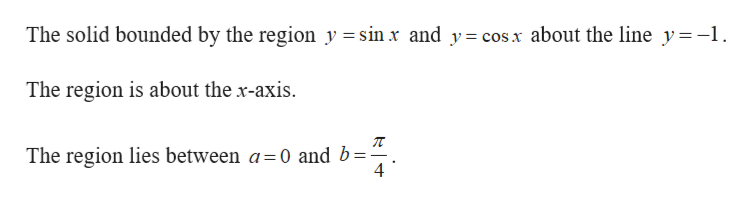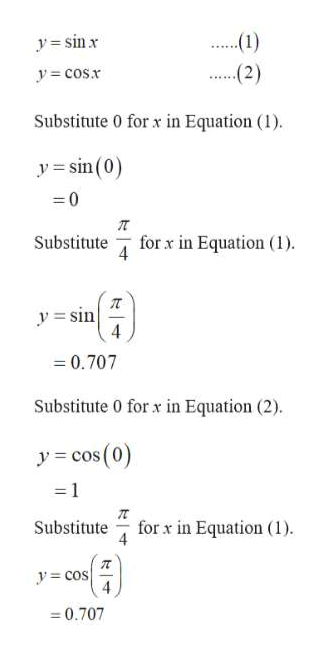Question
40 views

#14

check_circle

Step 1

Given:help_outlineImage TranscriptioncloseThe solid bounded by the region y =sin x and y= cos x about the line y-1 The region is about the x-axis. The region lies between a=0 and b= 4 fullscreen
Step 2

Sketch the Equation of the solid region.help_outlineImage Transcriptionclose1) y=sinx ..2) y cosx Substitute 0 for x in Equation (1) y sin(0) 0 for x in Equation (1) Substitute ysin 0.707 Substitute 0 for x in Equation (2) (0) ycos - 1 for x in Equation (1) Substitute y cos 4 0.707 fullscreen
Step 3

Sketch the region y = sin x,  y = cos x and  y = –1 usin...

### Want to see the full answer?

See Solution

#### Want to see this answer and more?

Solutions are written by subject experts who are available 24/7. Questions are typically answered within 1 hour.*

See Solution
*Response times may vary by subject and question.
Tagged in

### Integration